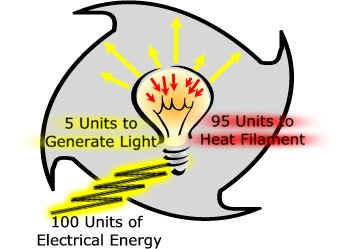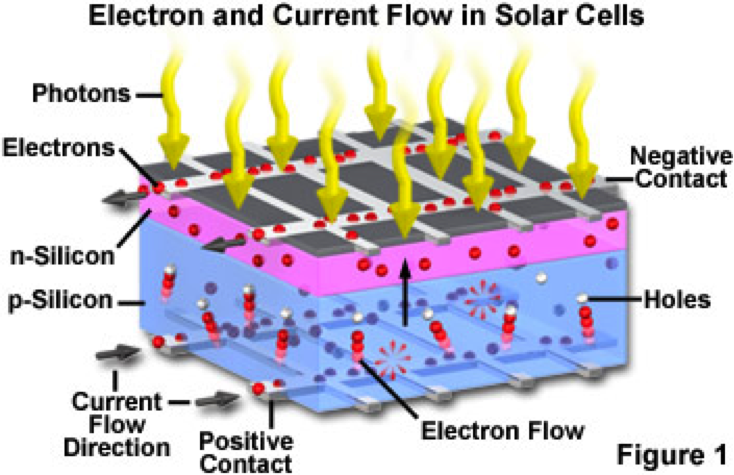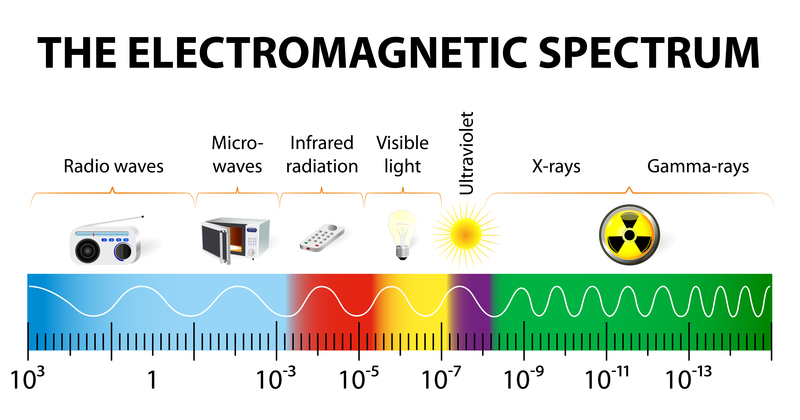As with any topic, it helps to know the basics. Let's learn how solar energy works.

### The First and Second Laws of Thermodynamics

The First Law of Thermodynamics 
The following statements are various ways of expressing the first law of thermodynamics:

• Energy is conserved.
• The amount of energy in the universe is constant.
• Energy can be neither created nor destroyed.
• There is no free lunch.
• It is impossible to build a machine that produces more energy than it uses (This type of machine is called a perpetual motion machine of the first kind.)

The Second Law of Thermodynamics
The following statements are various ways of expressing the second law of thermodynamics:

• With each energy conversion from one form to another, some of the energy becomes unavailable for further use.
• Heat cannot flow from a cold object to a hot object on its own.
• It is impossible to convert heat energy into work with 100 percent efficiency.
• You cannot break even.
• It is impossible to build a machine that produces as much energy as it uses. (This type of machine is called a perpetual motion machine of the second kind.)
• The entropy of the universe tends to a maximum. (Rudolf Clausius, 1865)

The First Law is saying all energy in the universe is saved or constant, that when energy is converted from one form to another there is no net loss of energy within the universe.

The Second Law is saying that even though energy is constant within the universe, when energy is converted from one form to another there is a net loss of energy in the converted form of energy. The energy that has been lost in the conversion is lost to the environment so that the net energy in the universe remains constant.

A good example of this is how an old fashioned incandescent light bulb works. When you flip the switch electricity flows across a wire filament within the bulb, heating the filament to the point that light energy is released.

100 units of electrical energy cannot be converted to 100 units of light energy. Instead, of the 100 units that are used to 
generate light, 95 are needed to heat the filament.The useful energy we are interested in is the light energy. It takes 100 units of electrical energy to produce those 5 units of light energy. The 95 units are lost to the environment as heat.

The Second Law of Thermodynamics is a key reason the abundant energy from the Sun is not easily translated into abundant electricity.

This is not only a problem for Solar energy. Every energy option is hamstrung by The Second Law of Thermodynamics. Fossil fuels get around this limitation by the fact they have accumulated over millions of years.

Let's learn more on exactly how Solar energy goes about converting the Sun's energy into useful energy - electricity.

### The Photovoltaic Effect - Photovoltaics (PV)

There are a few ways to convert the Sun's energy into electricity, but the Photovoltaic Effect is by far the most pervasive.

Photovoltaics is the direct conversion of light into electricity at the atomic level. Some materials exhibit a property known 
as the Photoelectric Effect that causes them to absorb photons of light and release electrons. When these free electrons are captured, an electric current results that can be used as electricity.

Silicon, by and large, is the material used for Photovoltaics, and the electricity produced is from the electrons in Silicon rather than direct Sun energy. When we get electricity from Photovoltaics, we are in fact capturing a small fraction of the Sun's energy.

Let's dig deeper into how Photovoltaics works.

### The PN Junction

In a very simple sense, the electricity being produced in a solar cell is from the Sun's photons knocking electrons free from the Silicon atoms and capturing those electrons to form an electric current. The key structure of a solar cell that accomplishes this task is the PN Junction.

The PN Junction is two layers of Silicon - one negatively charged and one positively charged - laid back to back. An electric field is created where the P layer and the N layer meet.

Other elements, such as phosphorous and boron, are added to Silicon to produce the N and P layers. These added elements are called impurities or doping agents. Silicon has 4 electrons in its outer shell. Phosphorous has 5 electrons in its outer shell, creating N Type Silicon, while Boron has 3 electrons in its outer shell, creating P Type Silicon.### Electromagnetic Spectrum and Band Gap Energy

There is an interesting relationship between the Sun's photons and the electrons of a particular material, as well as the strength of the PN Junction electric field in a particular material.

The Electromagnetic Spectrum is the range of energy within the universe in the form of waveforms (note: waveforms are dually represented as particles with specific energy levels), from long waveforms such as radio waves, to the visible light spectrum, all the way to the shortest waveforms such as gamma rays.Sunlight is composed of a spectrum of photons, and each photon is associated with a particular wavelength and hence also a particular energy level.

When sunlight shines on a Silicon Photovoltaic cell, not all photons are knocking lose electrons, only photons of a particular energy level are sufficient to knock a Silicon electron free. This characteristic of Silicon (and any material that has the photo electric effect) is called its Band Gap Energy.

The Band Gap Energy of crystalline silicon is 1.1 eV. A photon must contain 1.1 eV to knock 1 Silicon electron free. This means that any photon below that energy level will pass right through without producing any electricity, and it also means that the energy level of any photon above 1.1 eV will be wasted.

Why not find a material with low-enough band gap energy so we capture some energy from all of the photons in the electromagnetic spectrum? The catch is that a material with a lower band gap will also have a lower electric field force at the PN Junction. And this is a problem because,

The electron flow provides the current, and the cell's electric field causes a voltage. With both current and voltage, we [6,7]
have power, which is the product of the two.

P=VI

Power(watts) = Voltage(volts) x Current(amperes)

A material with a lower Band Gap Energy would produce more electrons or Current, but it would also have a lower Voltage, resulting in no net gain in Power.

In summary, the reason we can not easily convert the Sun's abundant energy into abundant electricity is due to The Second Law of Thermodynamics. Most of the Sun's photons are either passing right through the photovoltaic cell or a portion of their energy (anything above 1.1eV) is being lost to the environment. In fact, 70%  of the Sun's energy is lost in the photovoltaic production of electricity.

Next, we'll explore Solar energy efficiency in more detail. Is Solar energy performance improving? Will it act like semiconductors with ever-increasing computing power, and wow us with ever-increasing amounts of electricity in the same footprint?

Next: Solar Efficiency >